# How to Calculate and Solve for Emitter Discharge | Irrigation Water Requirement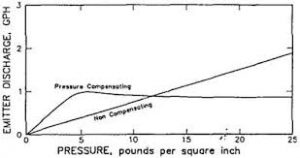The image above represents emitter discharge.

To compute for emitter discharge, three essential parameters are needed and these parameters are Constant for Each Emitter (K), Pressure Head (h) and Emitter Discharge Exponent (x).

The formula for calculating emitter discharge:

q = Khx

Where:

q = Emitter Discharge
K = Constant for Each Emitter
x = Emitter Discharge Exponent

Let’s solve an example;
Find the emitter discharge when the constant for each emitter is 21, the pressure head is 12 and the emitter discharge exponent is 2.

This implies that;

K = Constant for Each Emitter = 21
h = Pressure Head = 12
x = Emitter Discharge Exponent = 2

q = Khx
q = (21)(12)2
q = (21)(144)
q = 3024

Therefore, the emitter discharge is 3024.

Nickzom Calculator – The Calculator Encyclopedia is capable of calculating the emitter discharge.

To get the answer and workings of the emitter discharge using the Nickzom Calculator – The Calculator Encyclopedia. First, you need to obtain the app.

You can get this app via any of these means:

To get access to the professional version via web, you need to register and subscribe for NGN 2,000 per annum to have utter access to all functionalities.
You can also try the demo version via https://www.nickzom.org/calculator

Apple (Paid) – https://itunes.apple.com/us/app/nickzom-calculator/id1331162702?mt=8
Once, you have obtained the calculator encyclopedia app, proceed to the Calculator Map, then click on Agricultural under Engineering.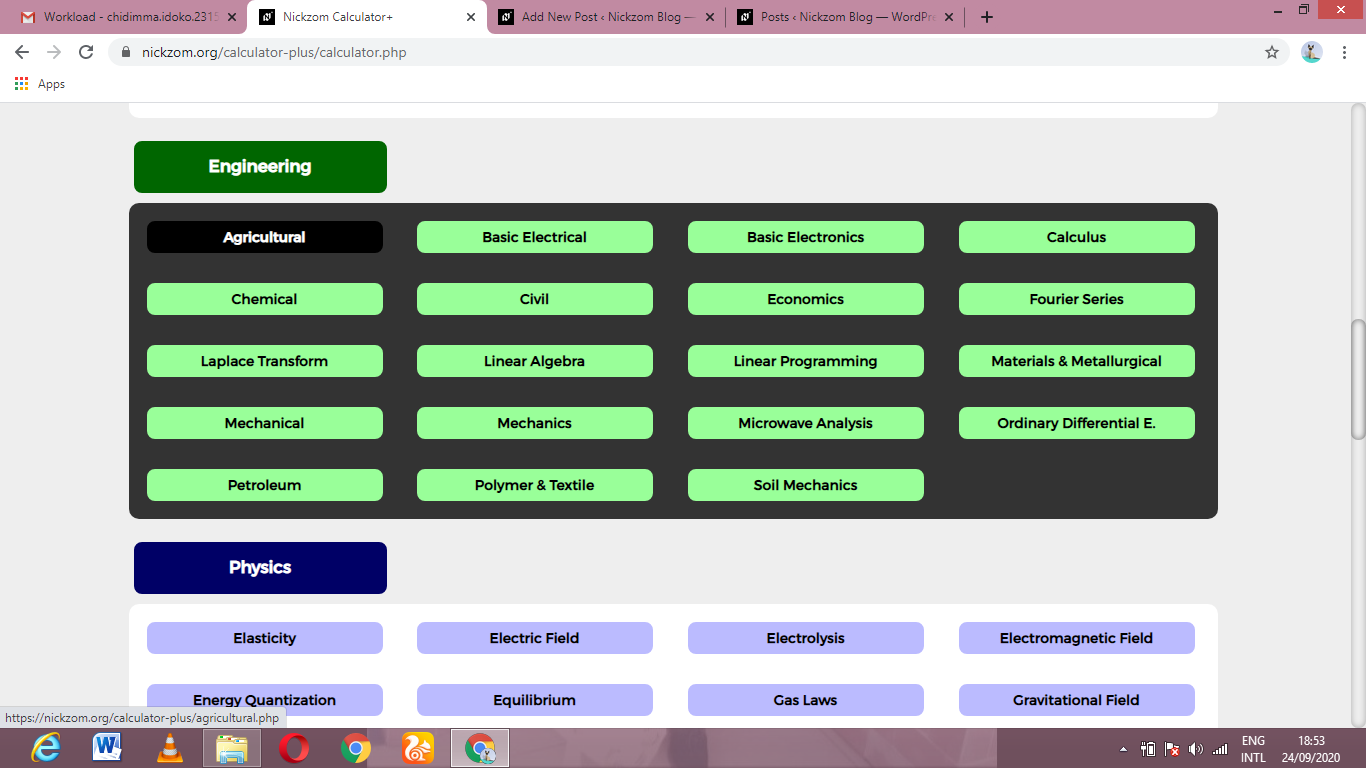Now, Click on Irrigation Water Requirement under AgriculturalNow, Click on Emitter Discharge under Irrigation Water RequirementThe screenshot below displays the page or activity to enter your values, to get the answer for the emitter discharge according to the respective parameters which is the Constant for Each Emitter (K), Pressure Head (h) and Emitter Discharge Exponent (x).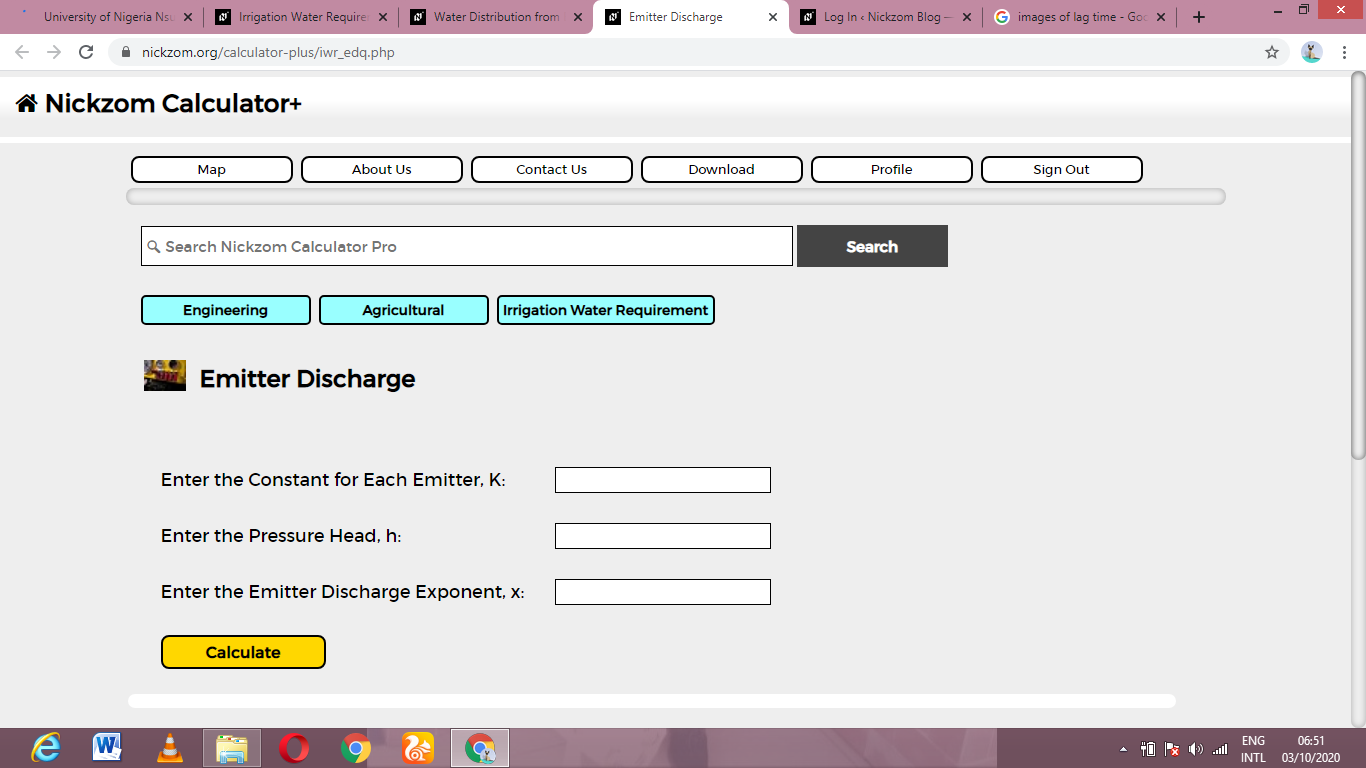Now, enter the values appropriately and accordingly for the parameters as required by the Constant for Each Emitter (K) is 21, Pressure Head (h) is 12 and Emitter Discharge Exponent (x) is 2.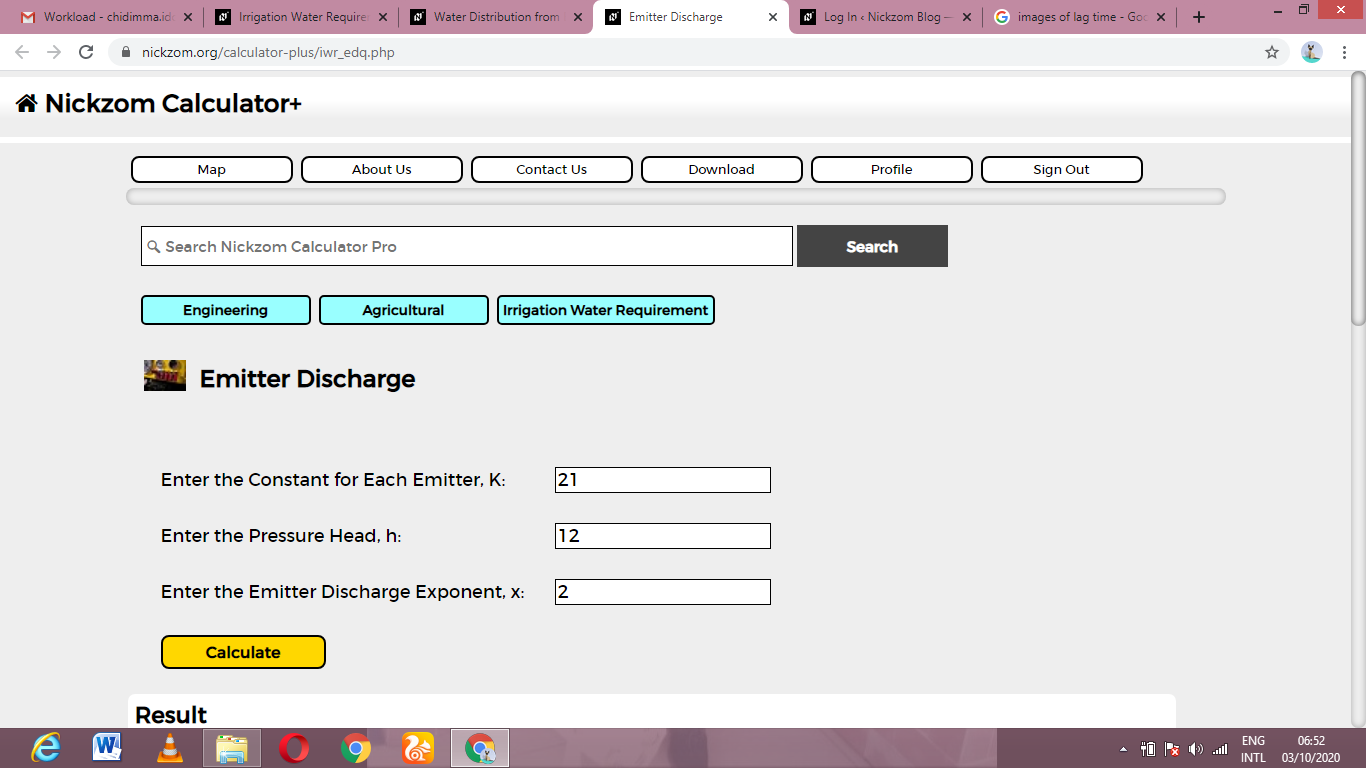Finally, Click on Calculate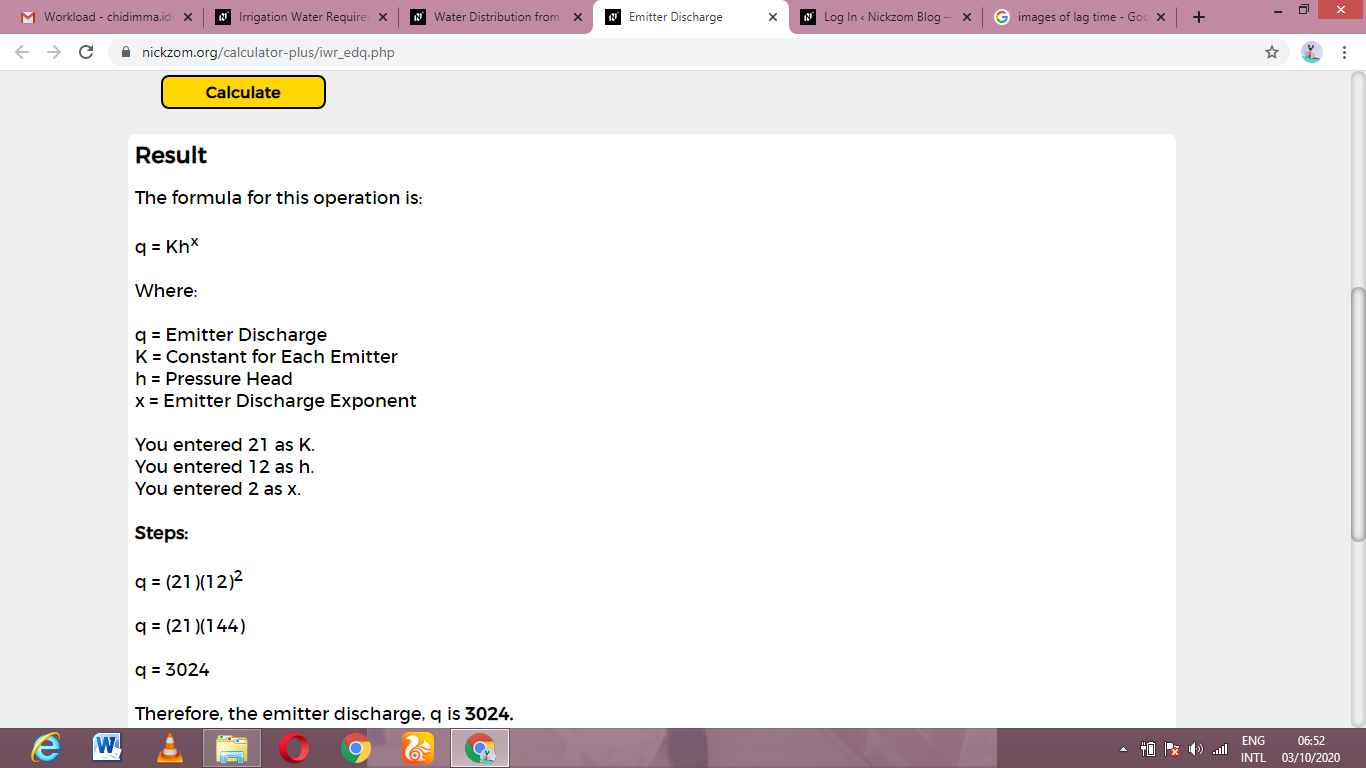As you can see from the screenshot above, Nickzom Calculator– The Calculator Encyclopedia solves for the emitter discharge and presents the formula, workings and steps too.